IF YOU CAN SEE THIS go to /sfa/Nuprl/Shared/Xindentation_hack_doc.html

### Multi-Argument Functions - continued from Function Types and Functions

Functions of more than one argument can be presented in various ways. The most common, of course, is to use an expression with free variables for the arguments.
But again, it is highly convenient to have closed forms of expression. We have not included a primitive type constructor for multi-argument functions. Instead, we use the method called "currying" in which A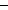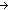BC is considered as a type of C-valued functions of two arguments, the first from type A and the second from type B. Note that fully parenthesized, "ABC" would be "A(BC)" and not "(AB)C".

To apply f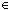ABC to two arguments, say aA and bB, first apply it to a, giving f(aBC, then apply that resulting function to b, giving f(a)(bC. We display this form of iterated application more conventionally as f(a,bC.

Naturally, the method generalizes to more argument places. If gABCD then g(a,b,cD, for aA, bB, cC.

Corresponding to the iteration of application, we can iterate the abstraction operation. Doing so assigns an order to the arguments.
For example, "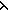x.y.x-y+2", which we may display as "x,yx-y+2", works like this when applied to a couple of arguments:

(x,yx-y+2)(3,6) *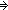(y.3-y+2)(6) *3-6+2 *-1

Or, we could assign a different order to the arguments when converting the free variable form of expression to the closed form:

(y,xx-y+2)(3,6) *(x.x-3+2)(6) *6-3+2 *5

We have been looking only at types for multi-argument functions where the output type is constant. The general form for the type of three-place functions, say, in which the output type may depend on the input values, and indeed, each input type may depend on values of earlier arguments, is "x:Ay:B(x)z:C(x;y)D(x;y;z)".
Suppose aA, bB(a), cC(a;b), and fx:Ay:B(x)z:C(x;y)D(x;y;z). Then

f(ay:B(a)z:C(a;y)D(a;y;z)

f(a,bz:C(a;b)D(a;b;z)

f(a,b,cD(a;b;c)

(Feb 2001 - sfa )